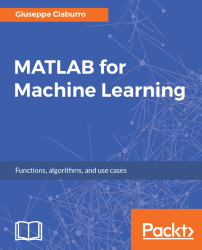•#### MATLAB for Machine Learning#### Overview of this book

MATLAB is the language of choice for many researchers and mathematics experts for machine learning. This book will help you build a foundation in machine learning using MATLAB for beginners. You’ll start by getting your system ready with t he MATLAB environment for machine learning and you’ll see how to easily interact with the Matlab workspace. We’ll then move on to data cleansing, mining and analyzing various data types in machine learning and you’ll see how to display data values on a plot. Next, you’ll get to know about the different types of regression techniques and how to apply them to your data using the MATLAB functions. You’ll understand the basic concepts of neural networks and perform data fitting, pattern recognition, and clustering analysis. Finally, you’ll explore feature selection and extraction techniques for dimensionality reduction for performance improvement. At the end of the book, you will learn to put it all together into real-world cases covering major machine learning algorithms and be comfortable in performing machine learning with MATLAB.
Title PageCreditsForewordwww.PacktPub.comCustomer FeedbackPrefaceFree Chapter
Getting Started with MATLAB Machine LearningImporting and Organizing Data in MATLABFrom Data to Knowledge DiscoveryFinding Relationships between Variables - Regression TechniquesPattern Recognition through Classification AlgorithmsIdentifying Groups of Data Using Clustering MethodsSimulation of Human Thinking - Artificial Neural NetworksImproving the Performance of the Machine Learning Model - Dimensionality ReductionMachine Learning in Practice## Working with media files

Images, videos, and audio files nowadays are an integral part of our lives. We use them widely thanks to the capabilities provided by modern devices that enable us to capture, store, and manipulate them. They often represent the input data we use in our machine learning applications, because through such algorithms, it is possible to extract a lot of information that at first glance is not so obvious. In this section, we will learn to deal with such files in the MATLAB environment.

### Handling images

Raster images are made up of a grid of colored pixels. In MATLAB, images can be represented as two-dimensional matrices, where each matrix element corresponds to a single pixel of the displayed image. For example, a 800x600 photo (consisting of 600 lines and 800 columns of pixel of different colors) will be stored as a 600x800 matrix. However, sometimes, a third dimension will be required to store the depth of color. For example, for RGB images, you will need to specify red...